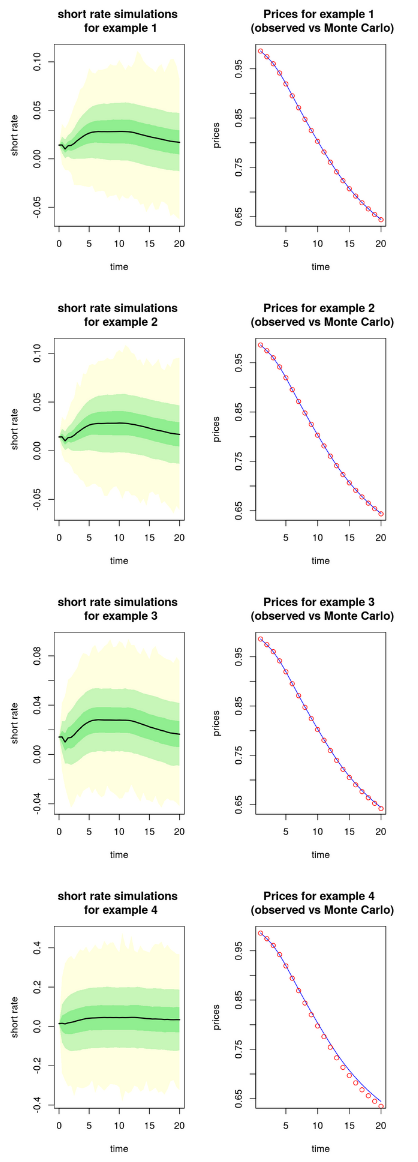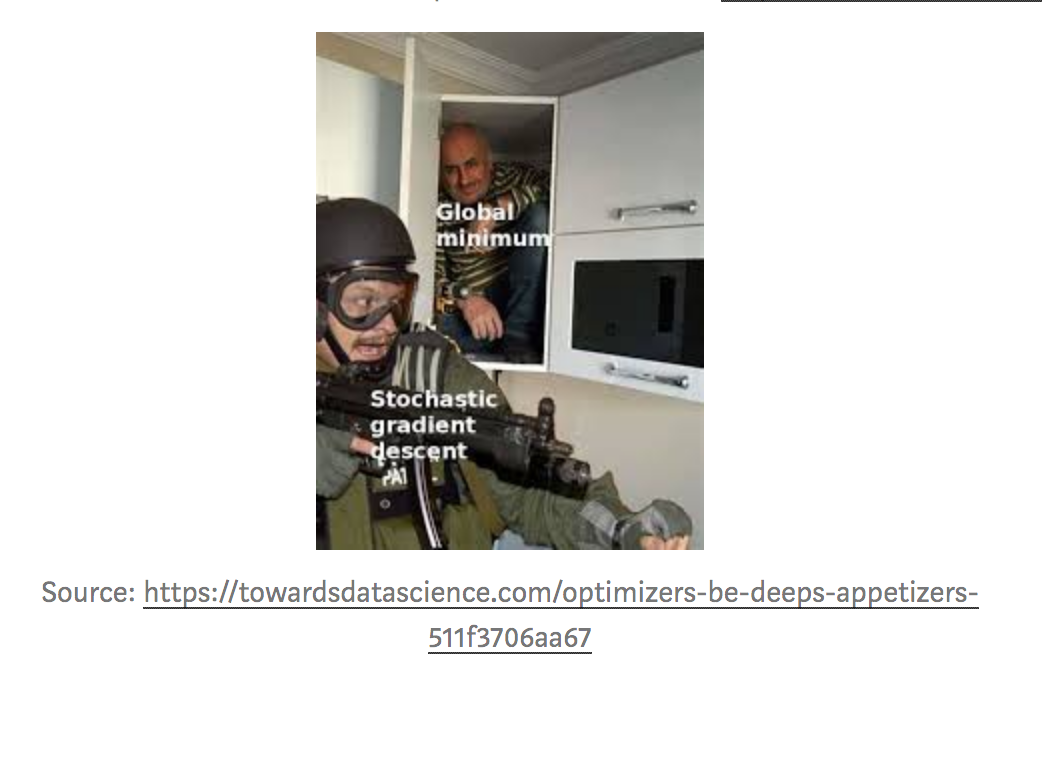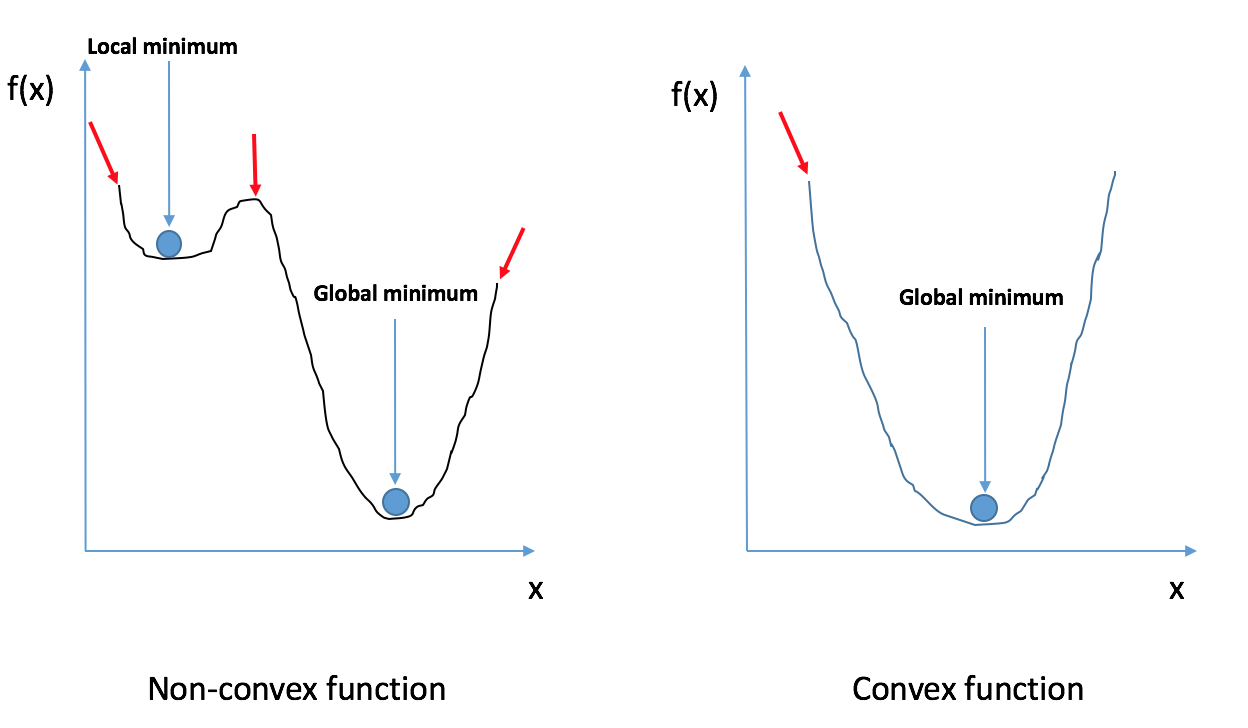I had a lot of fun this week, revisiting this blog post (Monte Carlo simulation of a 2-factor interest rates model with ESGtoolkit) I wrote a few years ago in 2014 – that somehow generated a heatwave. This 2020 post is about model specification, identification, degrees of freedom and regularization. The first part is on Monte Carlo simulation for financial pricing, and the second part on optimization in deep learning neural networks. I won’t draw a lot of conclusions here, but will let you draw your own. Of course, feel free to reach out if something seems/sounds wrong to you. That’s still the best way to deal with issues.

### Simulation of a G2++ short rates model

Let’s start by loading ESGtoolkit for the first part of this post:

# In R console
suppressPackageStartupMessages(library(ESGtoolkit))


G2++ Model input parameters:

# Observed maturities
u <- 1:30

# Yield to maturities
txZC <- c(0.01422,0.01309,0.01380,0.01549,0.01747,0.01940,
0.02104,0.02236,0.02348, 0.02446,0.02535,0.02614,
0.02679,0.02727,0.02760,0.02779,0.02787,0.02786,
0.02776,0.02762,0.02745,0.02727,0.02707,0.02686,
0.02663,0.02640,0.02618,0.02597,0.02578,0.02563)

# Zero-coupon prices = 'Observed' market prices
p <- c(0.9859794,0.9744879,0.9602458,0.9416551,0.9196671,
0.8957363,0.8716268,0.8482628,0.8255457,0.8034710,
0.7819525,0.7612204,0.7416912,0.7237042,0.7072136
,0.6922140,0.6785227,0.6660095,0.6546902,0.6441639,
0.6343366,0.6250234,0.6162910,0.6080358,0.6003302,
0.5929791,0.5858711,0.5789852,0.5722068,0.5653231)


G2++ simulation function (HCSPL stands for Hermite Cubic Spline interpolation of the Yield Curve):

# Function of the number of scenarios
simG2plus <- function(n, methodyc = "HCSPL", seed=13435,
b_opt=NULL, rho_opt=NULL, eta_opt=NULL,
randomize_params=FALSE)
{
set.seed(seed)

# Horizon, number of simulations, frequency
horizon <- 20
freq <- "semi-annual"
delta_t <- 1/2

# Parameters found for the G2++
a_opt <- 0.50000000 + ifelse(randomize_params, 0.5*runif(1), 0)
if(is.null(b_opt))
b_opt <- 0.35412030 + ifelse(randomize_params, 0.5*runif(1), 0)
sigma_opt <- 0.09416266
if(is.null(rho_opt))
rho_opt <- -0.99855687
if(is.null(eta_opt))
eta_opt <- 0.08439934

print(paste("a:", a_opt))
print(paste("b:", b_opt))
print(paste("sigma:", sigma_opt))
print(paste("rho:", rho_opt))
print(paste("eta:", eta_opt))

# Simulation of gaussian correlated shocks
eps <- ESGtoolkit::simshocks(n = n, horizon = horizon,
frequency = "semi-annual",
family = 1, par = rho_opt)

# Simulation of the factor x
x <- ESGtoolkit::simdiff(n = n, horizon = horizon,
frequency = freq,
model = "OU",
x0 = 0, theta1 = 0, theta2 = a_opt, theta3 = sigma_opt,
eps = eps[])

# Simulation of the factor y
y <- ESGtoolkit::simdiff(n = n, horizon = horizon,
frequency = freq,
model = "OU",
x0 = 0, theta1 = 0, theta2 = b_opt, theta3 = eta_opt,
eps = eps[])

# Instantaneous forward rates, with spline interpolation
methodyc <- match.arg(methodyc)
fwdrates <- ESGtoolkit::esgfwdrates(n = n, horizon = horizon,
out.frequency = freq, in.maturities = u,
in.zerorates = txZC, method = methodyc)
fwdrates <- window(fwdrates, end = horizon)

# phi
t.out <- seq(from = 0, to = horizon,
by = delta_t)
param.phi <- 0.5*(sigma_opt^2)*(1 - exp(-a_opt*t.out))^2/(a_opt^2) +
0.5*(eta_opt^2)*(1 - exp(-b_opt*t.out))^2/(b_opt^2) +
(rho_opt*sigma_opt*eta_opt)*(1 - exp(-a_opt*t.out))*
(1 - exp(-b_opt*t.out))/(a_opt*b_opt)
param.phi <- ts(replicate(n, param.phi),
start = start(x), deltat = deltat(x))
phi <- fwdrates + param.phi
colnames(phi) <- c(paste0("Series ", 1:n))

# The short rates
r <- x + y + phi
colnames(r) <- c(paste0("Series ", 1:n))

return(r)
}


Simulations of G2++ for 4 types of parameters’ sets:

r.HCSPL <- simG2plus(n = 10000, methodyc = "HCSPL", seed=123)

r.HCSPL2 <- simG2plus(n = 10000, methodyc = "HCSPL", seed=2020)

r.HCSPL3 <- simG2plus(n = 10000, methodyc = "HCSPL", seed=123,
randomize_params=TRUE)

r.HCSPL4 <- simG2plus(n = 10000, methodyc = "HCSPL", seed=123,
b_opt=1, rho_opt=0, eta_opt=0,
randomize_params=FALSE)


Stochastic discount factors derived from short rates simulations:

deltat_r <- deltat(r.HCSPL)

Dt.HCSPL <- ESGtoolkit::esgdiscountfactor(r = r.HCSPL, X = 1)
Dt.HCSPL <- window(Dt.HCSPL, start = deltat_r, deltat = 2*deltat_r)

Dt.HCSPL2 <- ESGtoolkit::esgdiscountfactor(r = r.HCSPL2, X = 1)
Dt.HCSPL2 <- window(Dt.HCSPL2, start = deltat_r, deltat = 2*deltat_r)

Dt.HCSPL3 <- ESGtoolkit::esgdiscountfactor(r = r.HCSPL3, X = 1)
Dt.HCSPL3 <- window(Dt.HCSPL3, start = deltat_r, deltat = 2*deltat_r)

Dt.HCSPL4 <- ESGtoolkit::esgdiscountfactor(r = r.HCSPL4, X = 1)
Dt.HCSPL4 <- window(Dt.HCSPL4, start = deltat_r, deltat = 2*deltat_r)



Prices (observed vs Monte Carlo for previous 4 examples):

# Observed market prices
horizon <- 20
marketprices <- p[1:horizon]

# Monte Carlo prices
## Example 1
montecarloprices.HCSPL <- rowMeans(Dt.HCSPL)
## Example 2
montecarloprices.HCSPL2 <- rowMeans(Dt.HCSPL2)
## Example 3
montecarloprices.HCSPL3 <- rowMeans(Dt.HCSPL3)
## Example 4
montecarloprices.HCSPL4 <- rowMeans(Dt.HCSPL4)


Plots observed prices vs Monte Carlo prices:


par(mfrow=c(4, 2))

ESGtoolkit::esgplotbands(r.HCSPL, xlab = 'time', ylab = 'short rate',
main="short rate simulations \n for example 1")
plot(marketprices, col = "blue", type = 'l',
xlab = "time", ylab = "prices", main = "Prices for example 1 \n (observed vs Monte Carlo)")
points(montecarloprices.HCSPL, col = "red")

ESGtoolkit::esgplotbands(r.HCSPL2, xlab = 'time', ylab = 'short rate',
main="short rate simulations \n for example 2")
plot(marketprices, col = "blue", type = 'l',
xlab = "time", ylab = "prices", main = "Prices for example 2 \n (observed vs Monte Carlo)")
points(montecarloprices.HCSPL2, col = "red")

ESGtoolkit::esgplotbands(r.HCSPL3, xlab = 'time', ylab = 'short rate',
main="short rate simulations \n for example 3")
plot(marketprices, col = "blue", type = 'l',
xlab = "time", ylab = "prices", main = "Prices for example 3 \n (observed vs Monte Carlo)")
points(montecarloprices.HCSPL3, col = "red")

ESGtoolkit::esgplotbands(r.HCSPL4, xlab = 'time', ylab = 'short rate',
main="short rate simulations \n for example 4")
plot(marketprices, col = "blue", type = 'l',
xlab = "time", ylab = "prices", main = "Prices for example 4 \n (observed vs Monte Carlo)")
points(montecarloprices.HCSPL4, col = "red")What do we observe on these graphs, both on simulations and prices? What will happen if we add a third factor to this model, meaning, three more parameters; a G3++/any other hydra?

### Optimization in Deep learning neural networks

On a different type of question/problem, but still on the subject of model specification, identification, degrees of freedom and regularization: Deep learning neural networks. Some people suggest that if you keep adding parameters (degrees of freedom?) to these models, you’ll still obtain a good generalization. Well, there’s this picture that I like a lot:When we optimize the loss function in Deep learning neural networks models, we are most likely using gradient descent, which is fast and scalable. Still, no matter how sophisticated the gradient descent procedure we’re using, we will likely get stuck into a local minimum – because the loss function is rarely convex.Stuck is a rather unfortunate term here, because it’s not an actual problem, but instead, an indirect way to avoid overtraining. Also, in our gradient descent procedure, we tune the number of epochs (number of iterations in the descent/ascent), the learning rate (how fast we roll in the descent/ascent), in addition to the dropout (randomly dropping out some nodes in networks’ layers), etc. These are also ways to avoid learning too much, to stop the optimization relatively early, and preserve the model’s ability to generalize. They regularize the model, whereas the millions of network nodes serve as degrees of freedom. This is a different problem than the first one we examined, with different objectives, but… still on the subject of model specification, identification, degrees of freedom and regularization.

For those who are working from home because of the COVID-19, I’d recommend this book about work-life balance, that I literally devoured a few months ago: REMOTE: Office Not Required (and nope, I’m not paid to promote this book).

Note: I am currently looking for a gig. You can hire me on Malt or send me an email: thierry dot moudiki at pm dot me. I can do descriptive statistics, data preparation, feature engineering, model calibration, training and validation, and model outputs’ interpretation. I am fluent in Python, R, SQL, Microsoft Excel, Visual Basic (among others) and French. My résumé? Here!